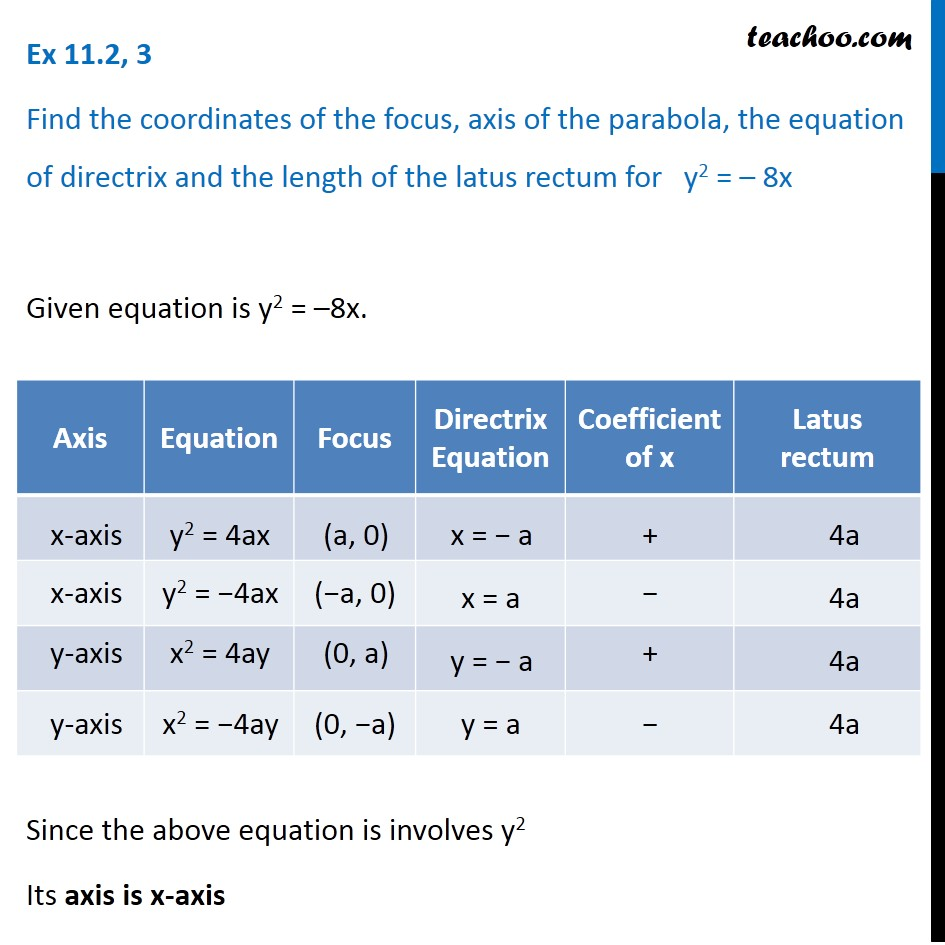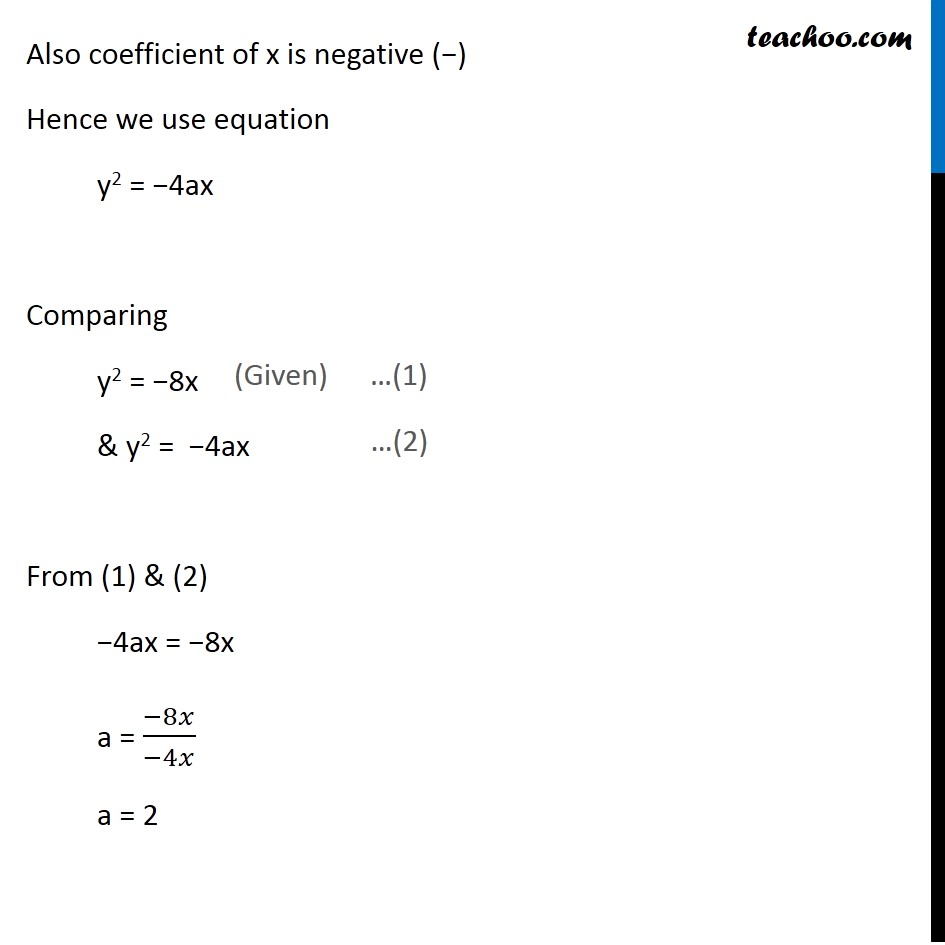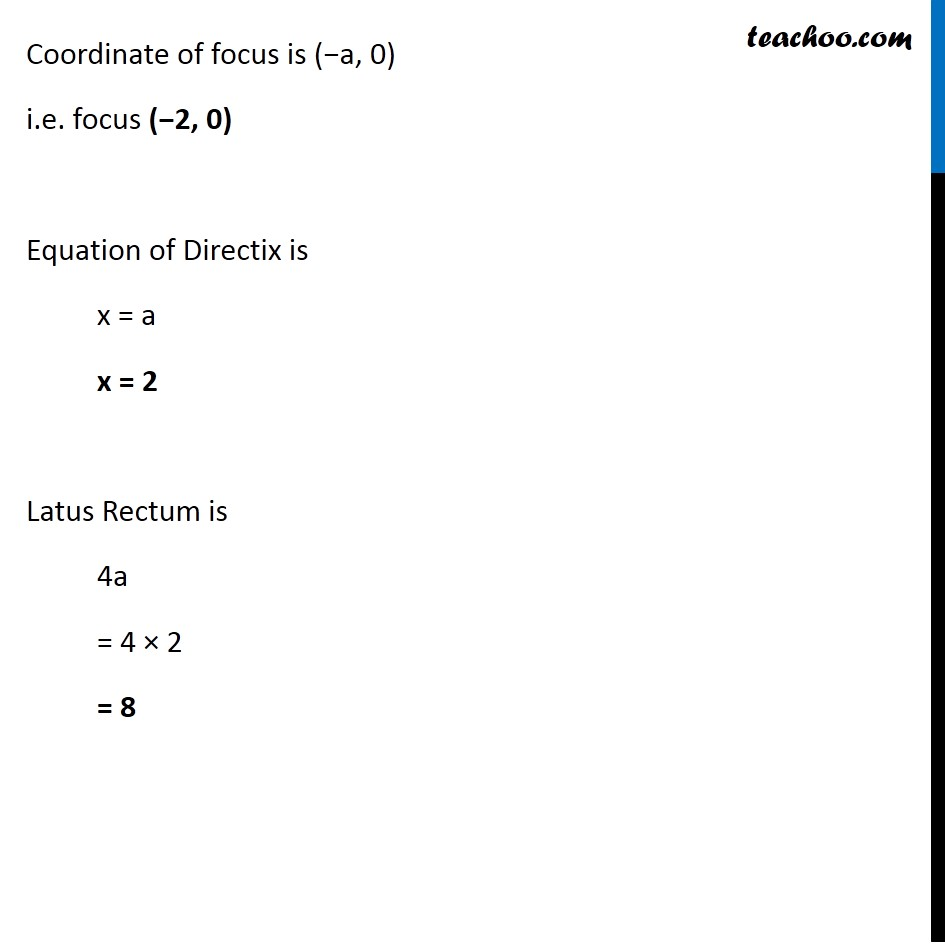Ex 10.2

Chapter 10 Class 11 Conic Sections
Serial order wiseLearn in your speed, with individual attention - Teachoo Maths 1-on-1 Class

### Transcript

Ex 10.2, 3 Find the coordinates of the focus, axis of the parabola, the equation of directrix and the length of the latus rectum for y2 = – 8x Given equation is y2 = –8x. Since the above equation is involves y2 Its axis is x-axis Also coefficient of x is negative (−) Hence we use equation y2 = −4ax Comparing y2 = −8x & y2 = −4ax From (1) & (2) −4ax = −8x a = (−8𝑥)/(−4𝑥) a = 2 Coordinate of focus is (−a, 0) i.e. focus (−2, 0) Equation of Directix is x = a x = 2 Latus Rectum is 4a = 4 × 2 = 8